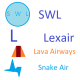Basic Waypoint System

3,220 EdamCheese6  7 days ago

This is for @PlaneFlightX mainly. The waypoint system is quite simple but there are a lot of ways you can improve it further. For my next build I am going to try and work in creating ground waypoints with the camera and having flight plans.Inputs

The main system uses a few custom button variables. If I started from scratch now, I would use one variable for all buttons with different output values. First, the numpad. This uses the input Num with interactionType set to Toggle. Each button has the outputValue set to the numerical value of that button, aside from 1 which has the default value of 1 and 0, which has the output of -1. Inside the global variable menu, create a variable called Num and on the right just put 0. This must be after all of the other variables we will create.

The minus symbol has a inputID of Neg, which too has a global variable setting it to a value of 0, which must also be after all other variables.

The WPT button has an input Waypoint, which has a setter to 0 at the end of the variables list.

The CLR button has an input of Clear, which has a setter of 0 at the end of the variable list.

The long and lat buttons have inputs LongUpdate and LatUpdate which also have setters to 0.

Getting Text Input

I have a variable which holds the currently entered number. To get each number to add, press the appropriate button on the keypad. This works with one variable because the output value is the same as the button digit. To store this value, I created a variable called NumInput. This variable can then be used to tranfer the number to other memory locations like waypoint coordinates. The funky trees code is below:

clamp((Num != 0 ? (NumInput*10+(Num = -1 ? 0 : Num)) : NumInput), -999999, 999999)*(1-Clear)*-1*(Neg*2-1)

The clamp statement can be removed if you want, it stops the input from being above or below a certain value. In my case it was to keep the number within the display. What is happening is that every time a key is pressed, the current input is multiplied by 10 and the new number is added. Since the default output of a non-pressed button is 0, then 0 cannot be the output value of the 0 button. So, I set the output value to be -1 and caught this with an IF statement. Then the value is multiplied by 1-Clear so when the CLR button is pressed the input is cleared. Last it is multiplied by -1*(Neg*2-1) which inverts the sign of the input. The only issue with this is that it has to be pressed after the first number since flipping the sign of 0 gives 0.

Setting Waypoint

My system currently only holds one waypoint. There are 2 main ways to set a waypoint. Pressing the WPT button creates a waypoint at the plane's current latitude and longitude. Pressing the Latitude Set or Longitude Set buttons replaces the current waypoint latitude or longitude with the current number input from NumInput.

The waypoint longitude and latitude are held in two variables. The first is tgtLong which has the expression Waypoint=1?Longitude:(LongUpdate=1?NumInput:tgtLong). This sets the waypoint longitude to the plane's longitude if the waypoint button is pressed, or to the number input if the Longitude Update button is pressed. If not it stays at its current value. The second variable is tgtLat, which works the same way. Waypoint=1?Latitude:(LatUpdate=1?NumInput:tgtLat)

Waypoint Data

The data stored for the waypoint is the heading to it, distance and 2D distance. This is because I assume all of the waypoints to have an altitude of 0, however you could create another variable like the one above so that you could input an altitude. The distance and 2D distance are calculated by pythagoras and stored in a variable. WaypointDist is set with sqrt(pow((tgtLat-Latitude),2)+pow((tgtLong-Longitude),2)+pow(Altitude, 2)). WaypointDist2D is the same without the altitude component. sqrt(pow((tgtLat-Latitude),2)+pow((tgtLong-Longitude),2)).
repeat(-((tgtLat-Latitude)>0?acos((tgtLong-Longitude)/WaypointDist2D):-acos((tgtLong-Longitude)/WaypointDist2D)+360)+450,360)

•21.2k PlaneFlightX

@EdamCheese6 Looks good, I'm making a prototype of this today. Thanks for the post.

5 days ago
•3,220 EdamCheese6

@PlaneFlightX I think that's everything. Let me know if I can help in any other ways.

5 days ago
•21.2k PlaneFlightX

@EdamCheese6 ah ok

+1 6 days ago
•3,220 EdamCheese6

@PlaneFlightX sorry, I'm In the UK so I've been asleep for like past few hours

6 days ago
•21.2k PlaneFlightX

Have you made any edits? It's said 'WIP' for a while.

+1 6 days ago
•21.2k PlaneFlightX

Tag me when it's finished pls (this post)

+2 7 days ago